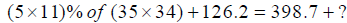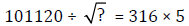Online Quiz for Quantitative AptitudeCourses

Q 1)

The smallest side of a right-angled triangle is 8 cm less than the side of a square of perimeter 56 cm. The second largest side of the right-angled triangle is 4 cm less than the length of the rectangle of area 96 sq cm and breadth 8 cm. What is the largest side of the right-angled triangle?

A) 20 cm

B) 12 cm

C) 10 cm

D) 15 cm

E) None of these

Q 2)A) 382

B) 422

C) 318

D) 428

E) None of these

Q 3)

A does 33.33% as much work as B in five-ninth of the time. They together do it in 15 day. C is 75% as efficient as B. Then A, B and C together can do the work in?

A) 7 days

B) 11 days

C) 10 days

D) 1010⁄47 days

E) None of these

Q 4)

(4+4×18−6−8)/(123×6−146×5) = ?

A) 7.75

B) 7.50

C) 8.84

D) 6.75

E) 5.50

Q 5)

A man takes thrice the time to cover upstream when compared to downstream. If the speed of stream is 6 km/hr, then what will be his speed in still water?

A) 8 kmph

B) 10 kmph

C) 12 kmph

D) 15 kmph

E) 16 kmph

Q 6)

A round table conference is to be held among 12 delegates. In how many ways can they be seated if two particular delegates are always to sit together?

A) 12!

B) 12! × 2!

C) 10! × 2!

D) 10!

E) None of these

Q 7)

What is the age of A and B?
I. Age of A is 80% of the age of C.
II. Age of B is 60% of the age of C.

A) If the question can be answered by using statement I alone but cannot be answered by statement II alone.

B) If the question can be answered by using statement II alone but cannot be answered by statement I alone.

C) If both statements I and II together are required to answer the question.

D) If the answer can be found by using any of the two statements alone.

E) If both the statements together are not sufficient to answer the question.

Q 8)

What is the percentage of students who scored more than or equal to 275 in all subjects?

A) 20%

B) 40%

C) 60%

D) 80%

E) None of these

Q 9)

Meenakshi started a business investing Rs. 12,000.After 5 months Abhay joined her with a capital of Rs. 9,000. At the end of the year the total profit was Rs. 4,416. What is the share of Meenakshi?

A) Rs. 1,344

B) Rs. 3,364

C) Rs.3,072

D) Rs. 2,568

E) None of these

Q 10)A) 4096

B) 64

C) 62

D) 3844

E) None of these